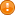Scilab Home page | Wiki | Bug tracker | Forge | Mailing list archives | ATOMS | File exchange
Change language to: English - Français - Português - Русский

See the recommended documentation of this function

Scilabヘルプ >> Elementary Functions > isequal

# isequal

comparison of objects

# isequalbitwise

comparison of objects (NaN are equal)

### Syntax

```t = isequal(a,b)
t = isequal(a,b,..)```

```t = isequalbitwise(a,b)
t = isequalbitwise(a,b,..)```

### Arguments

a, b , ...

objects: variable of any types ; expressions of any types of results

t

single boolean

### Description

`isequal` compares its arguments. If all of them are equal then the function returns `%t`. Otherwise it returns `%f`.

2 objects `a` and `b` are equal whether they fullfil ALL the following conditions:

• they must have the same type of containers: both are matrices or hypermatrices with the same data types, or lists, or cells arrays, or structures arrays, or any mlists or tlists of same types.
• they must have the same sizes.
• they must have the same encoding: boolean, int8, uint8, int16, uint16, int32, uint32, int64, uint64, decimal, complex, sparse
• If they are mlists or tlists such as structures, they must have the same fields in the same order.
• They must have the same contents, the same values at the same places.`1/%z == -1/(-%z)` returns `%T`, while `1/%z == 2/(2*%z)`, `isequal(1/%z, -1/(-%z))` and `isequal(1/%z, 2/(2*%z))` are `%F`.
If at least one of these conditions is not fulfilled, the objects are considered not equal.

When comparing nested heterogeneous containers like lists, structures, cells, etc, comparisons are performed recursively.With `isequal`, 2 compared Nan values are always considered not equal, according to the IEEE rule. On the opposite, with `isequalbitwise`, 2 compared Nan values are considered equal. This is the only difference between both functions.

### Examples

```// Examples returning %T
// =====================
a = [1 2];
isequal(a, [1 2])
isequal(a, 1)
isequal([1<2, %pi>%e], [3<10, %e>1])
isequal(-0, +0)
isequal([-%inf %inf], [-%inf %inf])
isequal(%z, poly(0,"z"), poly([0 1], "z", "coeff"))

// Functions "handles" can be compared:
s = sin;   isequal(s, sin)   // Built-in function
c = cosd;  isequal(c, cosd)  // Scilab function

// Comparisons are done recursively (here in nested lists):
L = list(%pi, "test", list(%t, 1/%z, 7), sin);
n = %pi; t = "test"; r = 1/%z; s = sin;
isequal(L, list(n, t, list(%t,r,7), s))

// ALL the following examples return %F
// ====================================
// 1) Containers must be identical:
isequal([1 %pi], {1, %pi})
isequal([1 %pi], list(1, %pi))

// 2) Encodings must be identical:
isequal([1 7], int8([1 7]))
isequal(uint8([1 7]), int8([1 7]))
isequal(int8([1 7]), int16([1 7]))

and([1 0]==[1 0*%i]) // is %T, but not as objects:
//isequal([1 0], [1 0*%i])  // bug http://bugzilla.scilab.org/9153#c12

p = (1+%z)^3
pc = p + 0*%i
p==pc
//isequal(p, pc)           // bug http://bugzilla.scilab.org/9153#c12

s = sprand(10,10,0.1); and(s==full(s)) // is %T, but not as objects:
isequal(s, full(s))

// 3) Sizes must be identical:
isequal([7 11], [7 11]')

// 4) fields of tlists or of mlists such as structures must be in the same order:
s1 = struct("r", %pi,     "t", "Hello")
s2 = struct("t", "Hello", "r", %pi)
//isequal(s1,s2)           // bug http://bugzilla.scilab.org/9153#c13

// Results with Nans
// =================
%nan==%nan                    // %F
isequal(%nan, %nan)           // %F
isequal([3 %nan], [3 %nan])   // %F
L = list(3, "test", %z, list(1, %nan, %T));
isequal(L,L)                  // %F
//isequalbitwise(L,L)           // %T ! bug http://bugzilla.scilab.org/14512```

• == — 比較, 関係演算子
• strcmp — 文字列を比較
• intersect — 2つのベクトルで共通の値のベクトルを返す
• assert_checkequal — 計算値と予測値が等しいことを確認する.
• assert_checkfilesequal — 2つのファイルが等しいことを確認する.